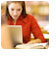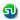### Quadratic Equation, real and equal roots problem. Quant Preparation. Ascent MBA TANCET, XAT, CAT, PGSEM Preparation, Correspondence course

 Home TANCET Classes GMAT Coaching Online GMAT Course CAT Classes TANCET Study Material
 TANCET '18 Classroom ProgramWeekend and weekday classes for TANCET MBA @ Chennai. At Nungambakkam and Velachery.Other Courses TANCET Correspondence Course CAT Classes & Courses GMAT Classes Chennai Online GMAT Course GRE Classes Chennai CBSE Math Online Tuition SAT Classes Other Links Ascent TANCET Toppers Testimonials CAT, TANCET Questions Careers @ Ascent Contact Us +91 44 4500 8484 +91 96000 48484 ascent@ascenteducation.com Postal Address Facebook / Twitter / Blog / Videos
You are here: Home  »  CAT, XAT, TANCET Prep Questions »  Quadratic Equation »   Question 8

# Math Problems : Quadratic Equation

## Question

For what value of m will the roots of the quadratic equation x2 + mx + 4 = 0 have real and equal roots?
1. 4
2. -4
3. 4 or -4
4. 16

Choice (3). 4 or -4

The question states that the roots of the equation are real and equal.

## Note

The roots of a quadratic equation of the form ax2 + bx + c = 0 will be real and equal if its discriminant D = b2 - 4ac = 0

In this case, b = m, a = 4 and c = 4.
Therefore, m2 - 4 * 4 * 1 = 0

Or m2 = 16

Orr m = +4 or m = -4.

## More Questions on Linear, Quadratic and Cubic Equations - Algebra

 5. 6. 7.

## CAT, XAT, TANCET Practice Questions and Answers : Listed Topicwise

 Number Theory Permutation Combination Probability Inequalities Geometry Mensuration Trigonometry Coordinate Geometry Percentages Profit Loss Ratio Proportion Mixtures Alligation Speed Time Distance Pipes Cisterns Interest Races Average, Mean AP, GP, HP Set Theory Clocks Calendars Algebra Function English Grammar General Awareness Data sufficiency TANCET Papers XAT PapersAdd to del.icio.usStumble Itdigg this